# Vector math techniques for scripting

I will be listing some methods for doing math with Vector3s, and explaining a bit about them. I am making this as a reference guide, so that people can quickly look up how to do something instead of giving themselves a headache trying to derive it lol. I’ll be writing them out in the form of functions for clarity, but you will mostly likely just want to copy paste what’s inside the function to use it.

This list is short for now, but I plan to add more methods as I think of them. Let me know if there’s anything you would like to know how to do and I’ll add them

How to create a vector that points along the direction of some vector

Details
``````function vec(length, referenceVec)
return length * referenceVec.Unit
end
``````

Explanation: Returns a vector that points in the direction of `referenceVec` and has a magnitude of `math.abs(length)`. A negative value of `length` will result in a vector pointing in the parallel but opposite direction of the reference vector.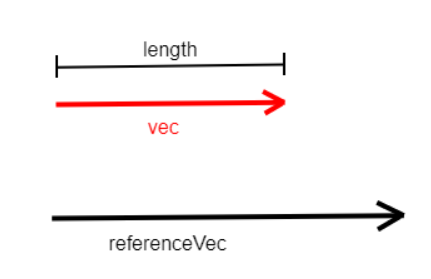How it works: `referenceVec.Unit` provides the same vector but with a magnitude of 1. It is then multiplied by `length` to stretch it to the desired magnitude (and possibly reflected).

Example use case: You want to launch a bullet out of the barrel of a gun at 1000 studs per second. The bullet’s velocity vector needs to point along the gun barrel and assuming the barrel is a cylinder part, its long axis is its X axis. Your velocity vector would be
`velocityVec = vec(1000, barrel.CFrame.XVector)`.

How to get the part of a vector that points parallel to some other vector

Details
``````function vec_para(vec, referenceVec)
return vec:Dot(referenceVec.Unit) * referenceVec.Unit
end
``````

Explanation: Given some reference vector, every vector can be split into the sum of a vector lying entirely along the reference vector, and a vector lying entirely perpendicular to the reference vector: `vec = vec_para + vec_perp`. This function returns the part parallel to the reference vector, `vec_para`. In math this would be called 'the projection of `vec` onto `referenceVec`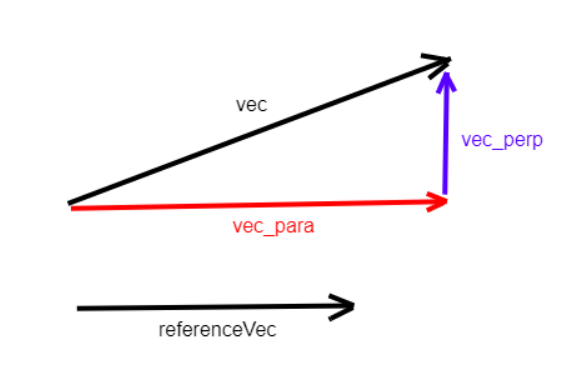How it works: `v1:Dot(v2)` is called the dot product of `v1` and `v2`. It multiplies the length of `v2` by the length of the part of `v1` that is parallel to `v2`. So `vec:Dot(referenceVec.Unit)` multiplies the length of `referenceVec.Unit` (which is 1) by the length of `vec` which is along that vector. Then multiplying this to `referenceVec.Unit` creates a vector which points along `referenceVec` with the length of the part of `vec` lying along `referenceVec`, which is exactly the projection of `vec` onto `referenceVec`.

Example use case: You are manually simulating a brick sliding down a ramp due to gravity (you don’t trust roblox physics). Only the part of gravity parallel to the surface of the ramp will work towards accelerating the brick so you need to find that part of of the gravity vector. The total acceleration of gravity is `gravityVec = workspace.Gravity * Vector3.yAxis`, and let’s call the vector pointing down the ramp `rampVec`. The brick’s net acceleration vector due to gravity is
`netAccelerationVec = vec_para(gravityVec, rampVec)`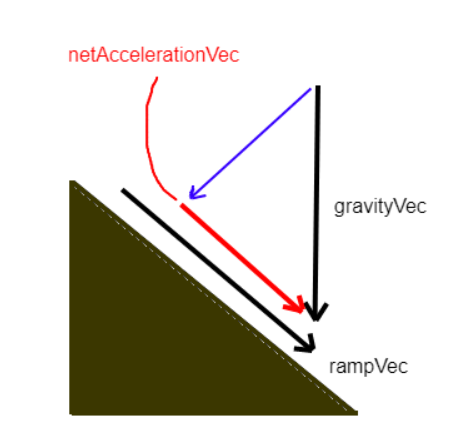How to get the part of a vector that points perpendicular to some other vector

Details
``````function vec_perp(vec, referenceVec)
return vec - vec:Dot(referenceVec.Unit) * referenceVec.Unit
end
``````

Explanation: As in the previous section, `vec` is treated as the sum of its parts parallel and perpendicular to `referenceVector`: `vec = vec_para + vec_perp`. This function provides the perpendicular part, `vec_perp`. In math this would be called 'the rejection of `vec` from `referenceVec`.How it works: Rearranging the above sum gives `vec_perp = vec - vec_para`. Then `vec_para` is replaced by its expression from the previous section: `vec_para = vec:Dot(referenceVec.Unit) * referenceVec.Unit`

Example use case: You want to do the same ramp simulation as in the previous example, but instead of a vector pointing along the surface of the ramp, you have a vector pointing out of the surface of the ramp, `rampNormalVec`. In this case the part of gravity along the ramp would be the rejection of the total gravity vector from this normal vector.
`netAccelerationVec = vec_perp(gravityVec, rampNormalVec)`

How to get the normal vector of a plane

Details
``````function normal(vec1, vec2)
return vec1:Cross(vec2).Unit
end
``````

Explanation: A plane is a 2 dimensional surface which can be defined by a vector called the ‘normal vector’ which is perpendicular to the plane and thus to every vector that lies in the plane. This function returns a unit normal vector of the plane when supplied with 2 independent vectors, `vec1` and `vec2`, that lie in the plane. Independent means the vectors can’t be parallel. Keep in mind that the order of `vec1` and `vec2` matters: reversing the order will reverse the direction of the normal (in the below image, vec1 then vec2 gives the + direction, and vec2 then vec1 gives the - direction. Right hand rule).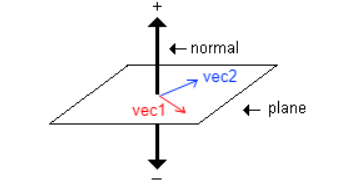How it works: For any 2 vectors `v1` and `v2`, `v1:Cross(v2)` is called the cross product of `v1` with `v2` and provides a vector which is perpendicular to both of them. The length of this vector is equal to the length of the rejection of `v1` from `v2`. For this function since `vec1` and `vec2` are vectors in the plane, `vec1:Cross(vec2)` is perpendicular to both of them and is therefore a normal vector to the plane. Only the direction of the normal matters, not the length, so it’s converted into a unit vector with `.Unit`.

Example use case: To find the part of a vector which lies in a plane, take the rejection of the vector from the plane’s normal. This is the part of the vector perpendicular to the normal so it is the part that lies in the plane. If the normal is found as `norm = normal(vec1, vec2)` then the part of some vector `vec` in the plane is
`vec_plane = vec - vec:Dot(norm) * norm`

Similarly, the part of `vec` which is perpendicular to the plane is found as the projection of `vec` onto the normal.
`vec_normal = vec:Dot(norm) * norm`

How to rotate a vector around a plane/about an axis

Details
``````function rotatedVec(vec, normalVec, angle)
return CFrame.FromAxisAngle(normalVec.Unit, angle) * vec
end
``````

Explanation: This takes the part of `vec` lying in the plane defined by the normal vector `normal` and rotates it counterclockwise (`normal` pointing out of the clock) around the plane by `angle`, while leaving the part of `vec` perpendicular to the plane alone. Equivalently, you can think of `normalVec` as the axis of rotation.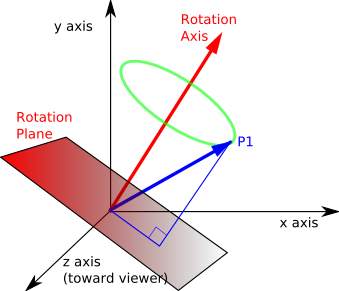How it works: `CFrame.fromAxisAngle(axis, angle)` creates a rotation matrix that rotates about the vector `axis` (in this application we used `normalVec`) by an amount given by `angle`. Multiplying this to `vec` gives the resultant rotated vector.

Example use case: You have a bunch of players sitting around a table and you want to shoot a random one with a ceiling mounted laser (using Raycast). To get the direction vector you start off with a vector pointing from the ceiling laser to one of the players `defaultDirection`. Then to randomize which seat the laser shoots at you rotate it about the Y axis by a random angle to get a randomized direction which still points towards a player.
`randomDirection = rotatedVec(defaultDirection, Vector3.Yaxis, 2*math.pi*math.random())`.
This vector would be used for the raycast direction vector.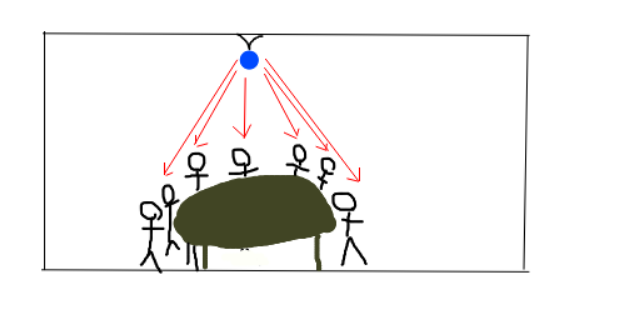How to find the angle between a vector’s projection onto a plane and some other vector’s projection onto that plane

Details
``````function angle(vec, referenceVec, normalVec)
local unitNorm = normalVec.Unit

local vec_p = (vec - vec:Dot(unitNorm) * unitNorm).Unit
local refVec_p = (referenceVec - referenceVec:Dot(unitNorm) * unitNorm).Unit

local cosine = vec_p:Dot(refVec_p)
local sine = vec_p:Dot(unitNorm:Cross(refVec_p))

return sine >= 0 and math.acos(cosine) or -math.acos(cosine)
``````

Explanation: This function projects both vectors onto the plane defined by `normalVec` and determines the angle that the `referenceVec` projection needs to be rotated around the plane by to point along the projection of `vec`

How it works: First, `normalVec` is converted to a unit vector for simpler calculation. Then the projections of `vec` and `referenceVec` onto the plane are found as the rejection from `unitNorm`. Since angle only depends on direction, the vectors are turned into unit vectors for simpler calculation. The result is `vec_p` and `refVec_p`. Then `vec_p` is decomposed into a right angle triangle with it’s adjacent side pointing along `refVec_p`. The cosine of this triangle is just the amount of `vec_p` along `refVec_p` and that is found with the dot product (if these were not unit vectors, the dot product would need to be divided by their magnitudes). The sine of the triangle is the length of `vec_p` perpendicular to `refVec_p` and that is found by taking the dot product of `vec_p` with the perpendicular vector found with `unitNorm:Cross(refVec_p)`. (The magnitude of rejection of `vec_p` from `refVec_p` can’t be used because the information of which side of `refVec_p` the sine is on is needed). `math.acos(cosine)` is used to extract the angle out of `cosine`. However there are 2 possible angles (one positive, one negative) which can return the same cosine. Which one is which depends on whether `sine` is negative or not, so the sign of `sine` is used to determine the correct angle.

Example use case: You have a player character, `patient`, on a surgery table and you need to amputate his left leg. Therefore you need to calculate the angle of his leg relative to the table so you know how to orient your scalpel to make the most precise cut. Assuming the scalpel is normally aligned with the -X axis of the table’s CFrame, that can be used as the reference vector `referenceVec`. Then the Y axis of the legs CFrame can be used as the target vector `vec`. And finally, the normal to the table is of course the Y axis of it’s CFrame. Then the angle needed to rotate the scalpel around the table surface is
`targetAngle = angle(patient.LeftUpperLeg.CFrame.YVector, -table.CFrame.XVector, table.CFrame.YVector)`

(Also amputate right leg for good measure)

31 Likes

Quite useful, but why such a gruesome example? lol

6 Likes

bro youve really broadened my knowledge here thanks a bunch man <33 great tutorial well done.

2 Likes

10 / 10 Tutorial, and 10 / 10 example.

1 Like